We think you are located in Nigeria. Is this correct?

# Don't get left behind

Join thousands of learners improving their maths marks online with Siyavula Practice.

# Chapter 9: Similar shapes

In this chapter, we will explore the mathematical meaning of the term "similar shapes". The general meaning of the word "similar" is that two or more things are almost the same. In Mathematics, similarity has a very specific meaning.

In Mathematics, two shapes are similar only if:

• their matching sides are in proportion, and
• their matching angles are equal.

In other words, if two objects are similar to each other, one of them can be "zoomed in" or "zoomed out" to make it identical to the other one.

similar Two shapes are similar only if their matching sides are in proportion, and their matching angles are equal.

## 9.1 Similar shapes around us

If you compare two different shapes in your environment, you will notice that some of the shapes are similar, and some of the shapes are not similar.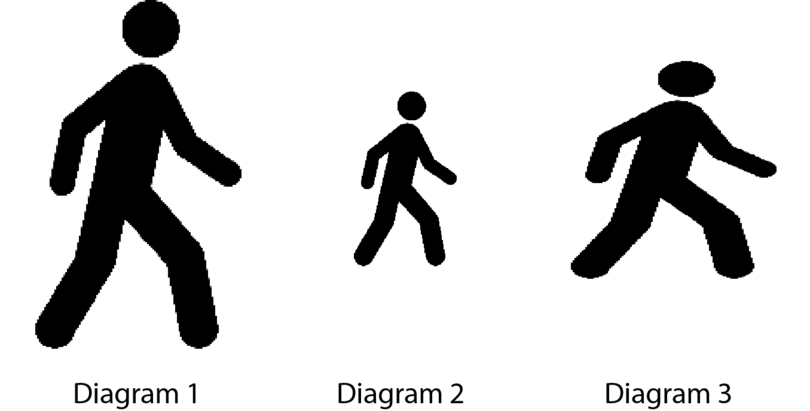The two figures in Diagram 1 and Diagram 2 are similar, because the figure has stayed in proportion in these two diagrams. The first figure is twice as tall and twice as broad as the second figure.

The figure in Diagram 3 is not similar to the figures in the other two diagrams, because the proportions are different. The relationships between the length and breadth of the third figure and the other two figures are different.

in proportion Two shapes are in proportion if all their dimensions are in the same ratio. For example, the length and breadth of B are three times the length and breadth of A, so A and B are in proportion.

ratio A ratio states what the relationship between two quantities or shapes is. For example, the ratio of A's length to B's length is $1 : 3$. We can also write ratio as a fraction, for example $\frac{1}{3}$.

### Exercise 9.1: Recognise similar shapes

1. Three diagrams of windows are given.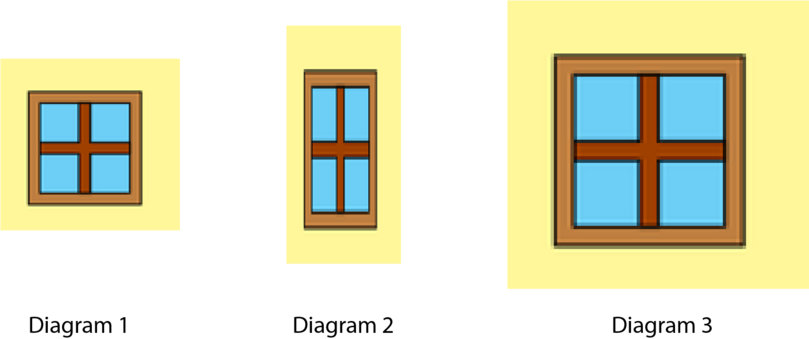Write down the numbers of the two diagrams that show similar windows.

The windows in Diagram 1 and Diagram 3 are similar.

2. Three diagrams of a shoe are given.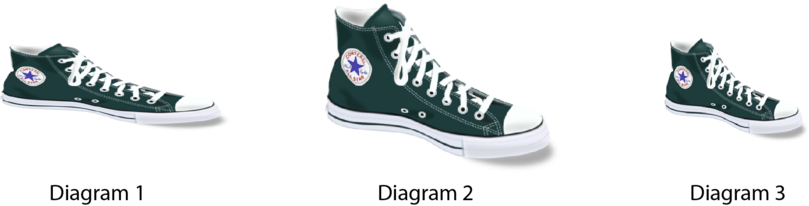Write down the numbers of the two diagrams that show similar shoes.

The shoes in Diagram 2 and Diagram 3 are similar.

3. The diagram below shows three pentagons.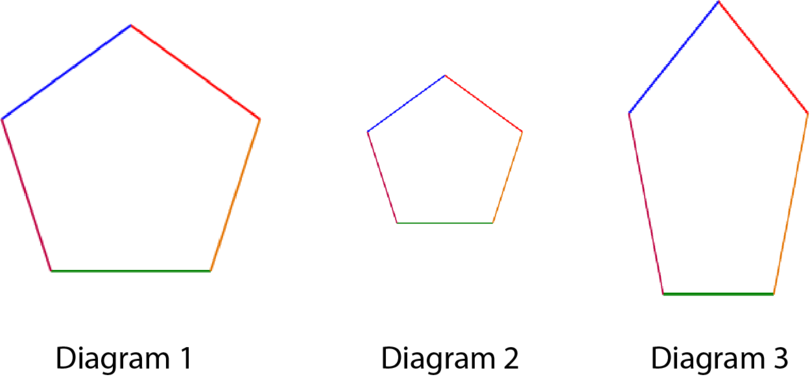Write down the numbers of the two diagrams that show similar pentagons.

The pentagons in Diagram 1 and Diagram 2 are similar.

4. The photo below shows cubes and plastic balls. Consider the photo and answer the questions that follow.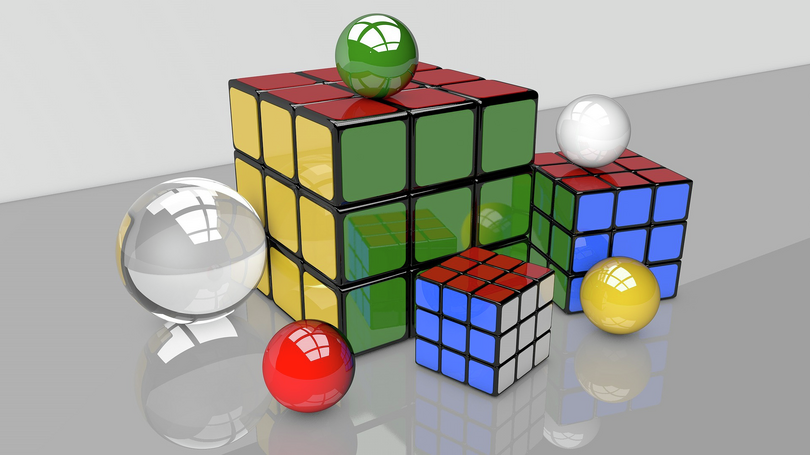1. True or false: The cubes are similar to each other.

True

1. True or false: The plastic balls are not similar to each other.

False. The plastic balls are similar to each other.

5. Explain why the pencils in the photo below are not similar.The pencils are not similar because all the pencils have the same thickness, but they are of different lengths.

For similar pencils, the shorter pencils would have to be thinner than the longer pencils.

### Similar shapes in Mathematics

We say that two shapes are similar if they are exactly the same shape, but one is bigger or smaller than the other one.

As we have seen, in Mathematics, two shapes are similar only if:

• their matching sides are in proportion, and
• their matching angles are equal.

In many polygons, we have to prove that the matching sides are in proportion and the matching angles are equal, if we want to be sure that the shapes are similar. But, in triangles, we only need to prove that one of them is true. This is a special property of triangles, which you will learn more about later in your school career.

Study the following three diagrams to learn more about what is meant when we say that two shapes are similar.

• The two kites shown below are not similar, because:
• their matching angles are equal, but
• their matching sides are not in proportion.
Kite $A$ Kite $B$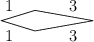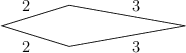• The two kites shown below are not similar, because:
• their matching sides are in proportion, but
• their matching angles are not equal.
Kite $A$ Kite $B$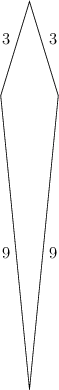• The two kites shown below are similar, because:
• their matching angles are equal, and
• their matching sides are in proportion.
Kite $A$ Kite $B$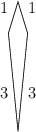### Exercise 9.2: Recognise similar shapes

1. In the photo below, a tiled wall is shown.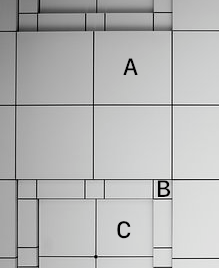Are the three tiles marked A, B and C similar? Give a reason for your answer.

Yes, tiles A, B and C are similar. They are all squares, and all squares are similar to each other because their matching angles are the same, and their matching sides are in proportion. Each square has four equal sides.

2. In the diagram below, two quadrilaterals are given.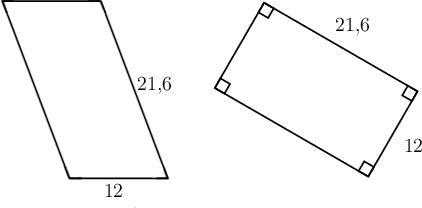Why are the two quadrilaterals not similar?

The matching angles of the two quadrilaterals are not the same.

3. The diagram below shows two triangles.The two triangles are similar:

• their matching angles are equal, and
• their sides are in proportion, because the measurements of the second triangle are three times bigger than the first triangle.
4. In the diagram below are two cuboids.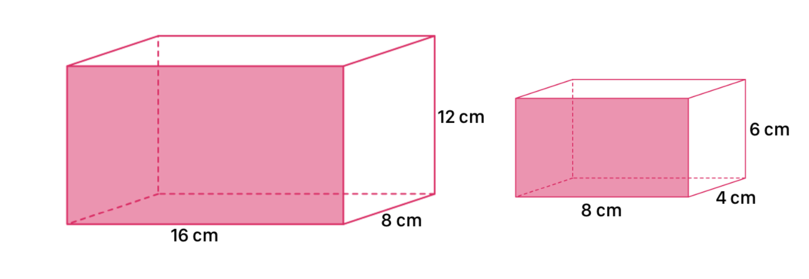The two cuboids are similar:

• the matching angles are equal, and
• the sides are in proportion, because the lengths of the second cuboid are all half the lengths of the first cuboid.
5. In the diagram below, two 3D objects are given.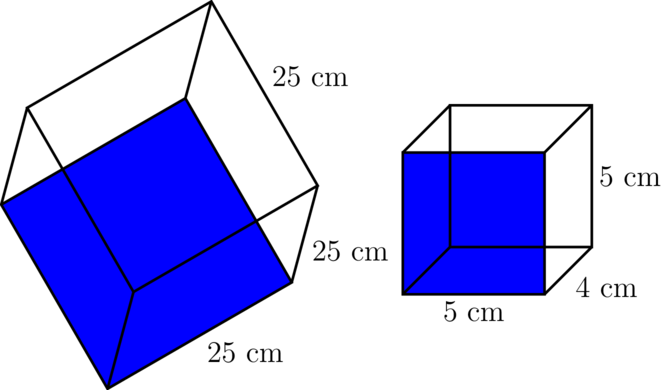Ekene says: "The two cubes in the diagram are similar, because all cubes are similar."

Do you agree with Ekene?

All cubes are similar, but only one of the objects in the diagram is a cube. The object with sides of 5 cm, 4 cm and 5 cm is not a cube.

Ekene's statement is incorrect.

## 9.2 Scale factors

The two kites drawn below are similar, because:

• their matching angles are equal, and
• their matching sides are in proportion.
Kite $A$ Kite $B$The sides of the two kites are in proportion. That means that the lengths of all the sides of one kite have been multiplied by the same number to get the lengths of the sides of the second kite.

If Kite $A$ is the given shape, all of the sides in kite $A$ have been multiplied by 2 to get the sides of Kite $B$.

Kite $B$ is the new shape.

We can write the lengths of the sides as fractions. Write the measurements of the new shape above the line and the measurements of the given shape below the line.

• The lengths of the longer sides as a fraction:
\begin{align} \dfrac{\text{longer side kite } B}{\text{longer side kite } A} & = \dfrac{10}{5} \\ & = 2 \\ \end{align}
• The lengths of the shorter sides as a fraction:
\begin{align} \dfrac{\text{shorter side kite } B}{\text{shorter side kite } A} & = \dfrac{4}{2} \\ & = 2 \\ \end{align}

In each case the answer is 2 .

Kite $B$ is 2 times bigger than kite $A$.

We say that kite $B$ is an enlargement of kite $A$ with a scale factor of 2.

We often use the variable $k$ to represent the scale factor, so in this example, $k = 2$.

When we write the lengths of sides as fractions, we always write the length of the new shape as the numerator (above the line) and the length of the given shape as the denominator (below the line).

enlargement An enlargement of a diagram, shape or object is a copy of the original in which everything is made larger, but keeping the same proportions.

scale factor The scale factor is the number by which every dimension of the given shape is multiplied to get the dimensions of the enlargement. We often use the variable $k$ to represent the scale factor.

### Worked example 9.1: Finding the scale factor

The diagram below shows two similar triangles: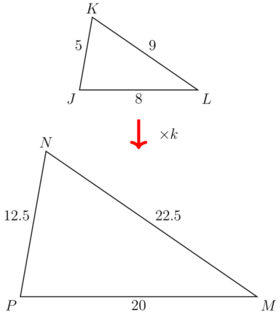Calculate the scale factor, $k$.

1. Step 1: Check that the two shapes are similar by finding the ratio of all the side lengths. Start by dividing the longest side in the new (bigger) triangle by the longest side in the given (smaller) triangle.

\begin{align} \frac{MN}{KL} & = \frac{22.5}{9} \\ &= \frac{22.5 \times 10}{9 \times 10} \\ &= \frac{225}{90} \\ & = 2.5\\ \end{align}
2. Step 2: Divide the shortest side in the new (bigger) triangle by the shortest side in the given (smaller) triangle.

\begin{align} \frac{PN}{JK} & = \frac{12.5}{5} \\ &= \frac{125}{50} \\ & = 2.5\\ \end{align}
3. Step 3: Divide the third side in the new (bigger) triangle by the third side in the given (smaller) triangle.

\begin{align} \frac{PM}{JL} &= \frac{20}{8} \\ &= 2.5 \\ \end{align}
4. Step 4: Make a conclusion.

The shapes are similar because comparing all the side lengths gives the same answer, which is 2.5.

This means that the new shape ( $\triangle PMN$) is 2.5 times bigger than the given shape ( $\triangle JKL$).

5. Step 5: Give the answer.

Remember that to divide by a decimal number, you need to multiply both the numerator and the denominator by the same power of 10 so that you can divide by a whole number.

For example:

\begin{align} \frac{20}{0.4} &= \frac{20 \times 10}{0.4 \times 10} \\ &= \frac{200}{4} \\ &= 50 \\ \end{align}

### Exercise 9.3: Find the scale factor

1. The diagram below shows two similar squares. The figures are drawn to scale. Find the value of $k$, the scale factor.

Shape $A$ Shape $B$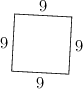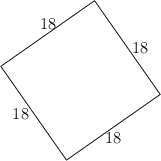\begin{align} \frac{\text{side of new square}}{\text{side of given square}} &= \frac{18}{9} \\ & = 2 \\ \end{align}
2. Two similar triangles are given. $\triangle XYZ$ is an enlargement of $\triangle PQR$.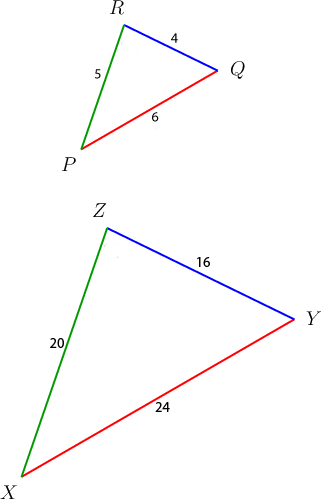Find the value of the scale factor, $k$.

Ratio of longest sides:

\begin{align} \frac{XY}{PQ} &= \frac{24}{6} \\ & = 4 \\ \end{align}

Ratio of shortest sides:

\begin{align} \frac{ZY}{RQ} &= \frac{16}{4} \\ & = 4 \\ \end{align}

Ratio of third sides:

\begin{align} \frac{XZ}{PR} &= \frac{20}{5} \\ & = 4 \\ \end{align}

All the fractions give 4 as the answer, so the shapes are similar, and the scale factor is 4.

3. In the diagrams below, rectangle $ABCD$ is an enlargement of rectangle $PQRS$.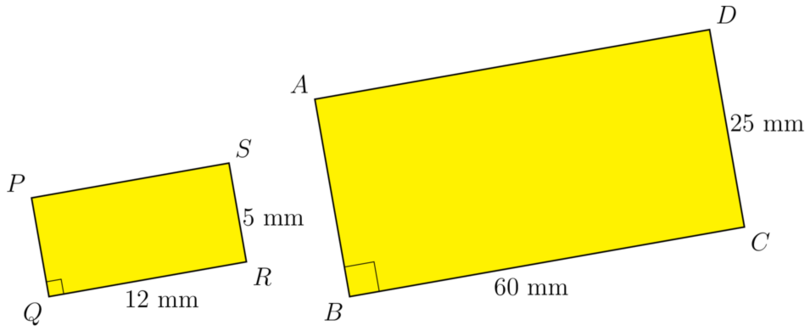Find the value of the scale factor, $k$.

Ratio of longer sides:

\begin{align} \frac{BC}{QR} &= \frac{60}{12} \\ & = 5 \\ \end{align}

Ratio of shorter sides:

\begin{align} \frac{DC}{SR} &= \frac{25}{5} \\ & = 5 \\ \end{align}
4. The diagrams below show quadrilateral $QLMP$, which is an enlargement of quadrilateral $JKNR$.Find the value of the scale factor, $k$.

Ratio of longest sides:

\begin{align} \frac{LM}{KN} &= \frac{15}{5} \\ & = 3 \\ \end{align}

Ratio of second longest sides:

\begin{align} \frac{QL}{JK} &= \frac{10.5}{3.5} \\ &=\frac{10.5 \times 10}{3.5 \times 10}\\ &= \frac{105}{35} \\ & = 3 \\ \end{align}

Ratio of shortest sides:

\begin{align} \frac{PM}{RN} &= \frac{6}{2} \\ & = 3 \\ \end{align}

Ratio of second shortest sides:

\begin{align} \frac{PQ}{JR} &= \frac{9}{3} \\ & = 3 \\ \end{align}
5. Two cubes are given. Consider the information and answer the questions that follow.
• Cube $S$ has sides each of length 7 cm.
• Cube $T$ has sides each of length 70 cm.
1. True or false: Cube $S$ is an enlargement of cube $T$.

The statement is false. Cube $T$ is an enlargement of cube $S$.

1. Calculate the value of the scale factor, $k$.
\begin{align} \frac{\text{side cube } T}{\text{side cube } S} &= \frac{70}{7} \\ & = 10 \\ \end{align}
6. Cuboid $B$ is an enlargement of cuboid $A$.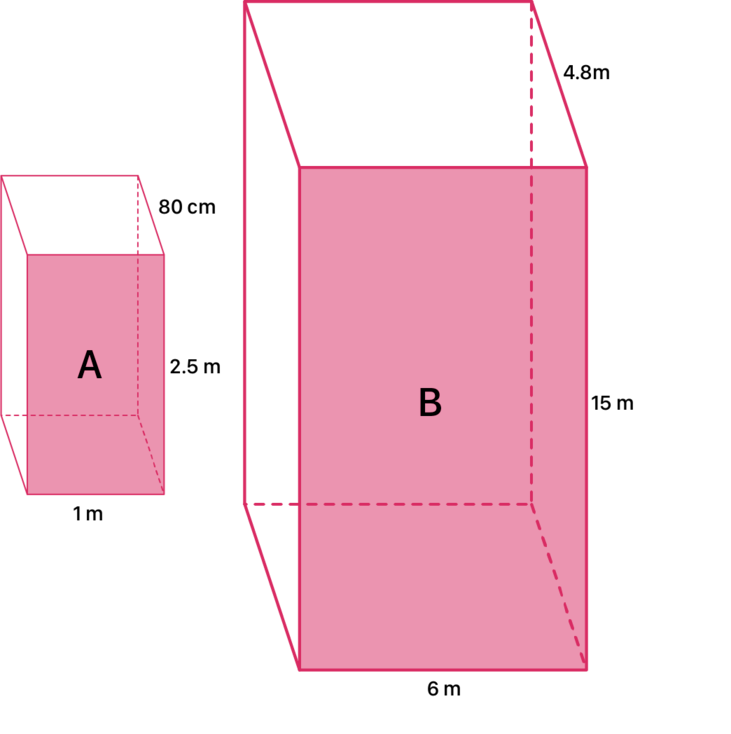Find the value of the scale factor, $k$.

Ratio of lengths:

\begin{align} \frac {\text{4.8 m}} {\text{80 cm}} &= \frac {\text{480 cm}} {\text{80 cm} }\\ & = \frac{480}{80} \\ & = 6 \\ \end{align}

Ratio of heights:

\begin{align} \frac{15}{2.5} & = \frac{15 \times 10}{2.5 \times 10} \\ & = \frac{150}{25} \\ & = 6 \\ \end{align}
7. Umar says $\triangle DEF$ is not an enlargement of $\triangle CAB$.Do you agree with Umar or not? Give a reason for your answer.

Ratio of longer sides:

\begin{align} \frac{DE}{AC} & = \frac{16}{3} \\ & =5 \frac{1}{3} \\ \end{align}

Ratio of shorter sides:

\begin{align} \frac{DF}{CB} & = \frac{12}{3} \\ & = 4 \\ \end{align}

The two ratios are not the same, so $\triangle DEF$ is not an enlargement of $\triangle CAB$. Umar's statement is correct.

8. The diagram below shows two triangles. $\triangle PQR$ is the given shape and $\triangle XYZ$ is the new shape.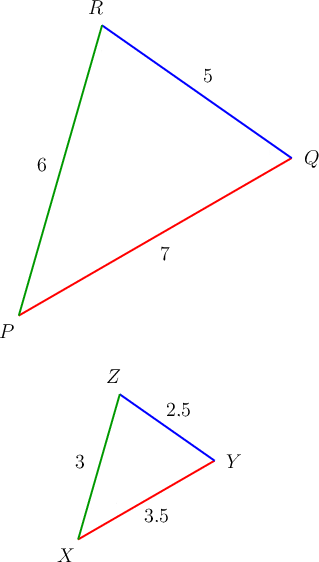Find the value of $k$, the scale factor.

Ratio of longest sides:

\begin{align} \frac{XY}{PQ} &= \frac{3.5}{7} \\ &= \frac{3.5 \times 10}{7 \times 10} \\ &= \frac{35}{70} \\ & = \frac{1} {2} \\ \end{align}

Ratio of shortest sides:

\begin{align} \frac{ZY}{RQ} &= \frac{2.5}{5} \\ &= \frac{2.5 \times 10}{5 \times 10} \\ &= \frac{25}{50} \\ & = \frac{1}{2} \\ \end{align}

Ratio of third sides:

\begin{align} \frac{XZ}{PR} &= \frac{3}{6} \\ & = \frac{1}{2} \\ \end{align}

An enlargement where the scale factor is a fraction between 0 and 1 leads to a new shape or object that is smaller than the given shape or object.

### Enlarging figures using scale factors

You know now that an enlargement is a larger version (or a smaller version) of an original length, shape or object. To ensure that the enlargement has the same proportions, we multiply each dimension by the same scale factor, represented by $k$.

If you are given the scale factor, you can calculate the dimensions of an enlargement of a given shape or object. To do this, you will use the equation given above in this form:

### Worked example 9.2: Using a scale factor

Rectangle $STUV$ is an enlargement of rectangle $ABCD$.Calculate the length and the breadth of rectangle $STUV$ if the scale factor is 3.

1. Step 1: Write down the rule to calculate the new length.

2. Step 2: Substitute the given values.

\begin{align} \text{length of new shape} & = k \times \text{length of given shape}\\ & = 3 \times \text{25 mm} \\ \end{align}
3. Step 3: Calculate the new length.

\begin{align} \text{length of new shape} & = k \times \text{length of given shape}\\ & = 3 \times \text{25 mm} \\ & = \text{75 mm}\\ \end{align}
4. Step 4: Write down the rule to calculate the new breadth.

5. Step 5: Substitute the given values.

\begin{align} \text{breadth of new shape} & = k \times \text{breadth of given shape}\\ & = 3 \times \text{12 mm} \\ \end{align}
6. Step 6: Calculate the new breadth.

\begin{align} \text{breadth of new shape} & = k \times \text{breadth of given shape}\\ & = 3 \times \text{12 mm} \\ & = \text{36 mm}\\ \end{align}

If the scale factor is 3, the length of rectangle $STUV$ is 75 mm and the breadth is 36 mm.

### Exercise 9.4: Use the scale factor

1. Squares $X$ and $Y$ are given. Square $Y$ is an enlargement of square $X$.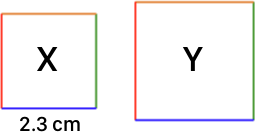Find the measurements of square $Y$ if the scale factor is 7.

\begin{align} \text{side of new square} & = k \times \text{side of given square}\\ & = 7 \times \text{2.3 cm} \\ & = \text{16.1 cm}\\ \end{align}

Square $Y$ has sides of 16.1 cm.

2. $\triangle DEF$ is an enlargement of $\triangle CBA$. Both triangles are right-angled triangles and $k = 2$.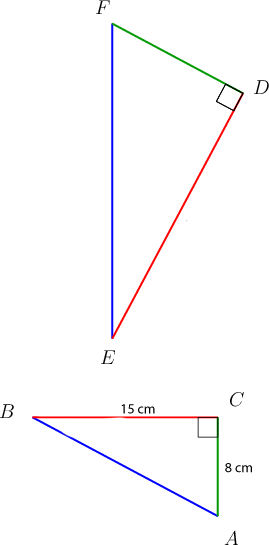Calculate the lengths of $AB$, $DE$, $EF$, $DE$ and $DF$.

You will need to use the theorem of Pythagoras, which states that in a right-angled triangle, $\text{(length of hypotenuse)}^2 = \text{(length of other two sides)}^2$

Use the theorem of Pythagoras to calculate the length of $AB$:

\begin{align} AB^{2} & = BC^{2} + AC^{2} \quad \text{(Pythagoras)} \\ & = 15^{2} + 8^{2} \\ & = 225 + 64 \\ & = 289 \\ \therefore AB & = \sqrt{289} \\ & = \text{17 cm} \\ \end{align}

Use the scale factor to calculate the length of $EF$:

\begin{align} EF & = k \times AB\\ & = 2 \times \text{17 cm} \\ & = \text{34 cm}\\ \end{align}

Use the scale factor to calculate the length of $DE$:

\begin{align} DE & = k \times BC\\ & = 2 \times \text{15 cm} \\ & = \text{30 cm}\\ \end{align}

Use the scale factor to calculate the length of $DF$:

\begin{align} DF & = k \times AC\\ & = 2 \times \text{8 cm} \\ & = \text{16 cm}\\ \end{align}

The measurements for the new $\triangle DEF$ are shown in the diagram below.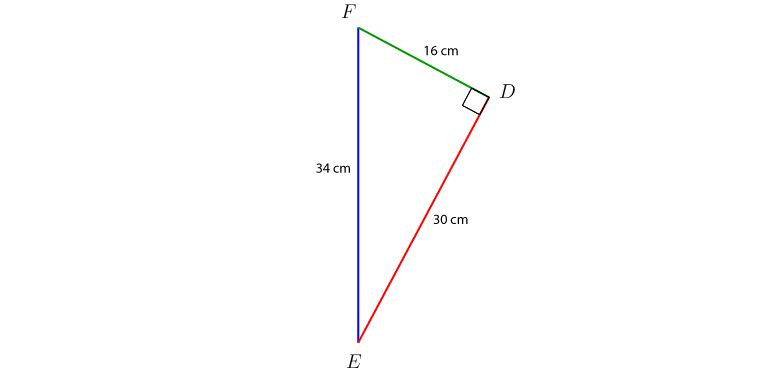3. In the diagram below, parallelogram $GCDH$ is an enlargement of parallelogram $ABEF$.Calculate the dimensions of parallelogram $GCDH$ if it is given that $k = 9$.

Use scale factor to calculate the length of the longer sides:

\begin{align} HD& = k \times FE\\ & = 9 \times \text{42 mm} \\ & = \text{378 mm}\\ \end{align}

Use scale factor to calculate the length of the shorter sides:

\begin{align} CD & = k \times BE\\ & = 9 \times \text{31 mm} \\ & = \text{279 mm}\\ \end{align}

The dimensions of parallelogram $GCDH$ are length 378 mm and breadth 279 mm.

4. The cube shown below has sides of 8.5 cm.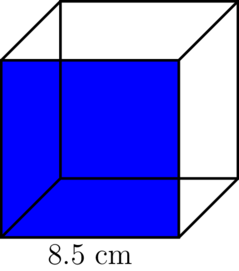Calculate the length of one side of the cube if the cube is enlarged by a scale factor of 4.

\begin{align} \text{new side} & = k \times \text{given side}\\ & = 4 \times \text{8.5 cm} \\ & = \text{34 cm}\\ \end{align}
5. A cuboid with the following dimensions are given:
• length = 40 cm
• height = 35 cm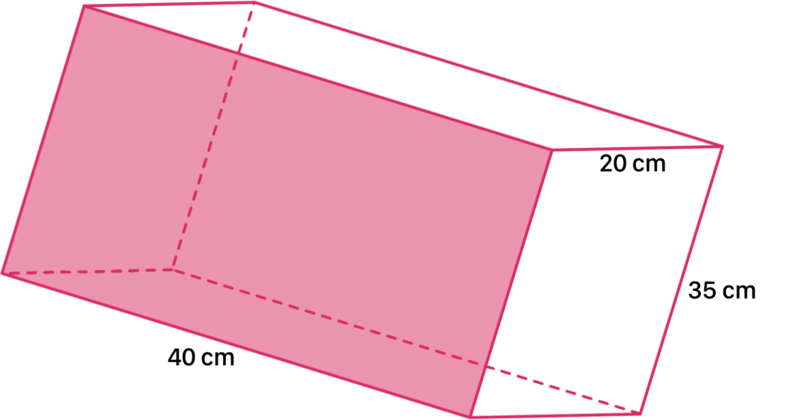Calculate the dimensions of the new cuboid that is an enlargement of the given cuboid. Use a scale factor of 1.5.

Length:

\begin{align} \text{new length} & = k \times \text{given length}\\ & = 1.5 \times \text{40 cm} \\ & = \text{60 cm}\\ \end{align}

\begin{align} \text{new breadth} & = k \times \text{given breadth}\\ & = 1.5 \times \text{20 cm} \\ & = \text{30 cm}\\ \end{align}

Height:

\begin{align} \text{new height} & = k \times \text{given height}\\ & = 1.5 \times \text{35 cm} \\ & = \text{52.5 cm}\\ \end{align}

The dimensions of the new cuboid are $60 \times 30 \times 2.5$ cm.

6. Polygon $H$ is an enlargement of polygon $G$. Consider the two polygons and answer the questions that follow.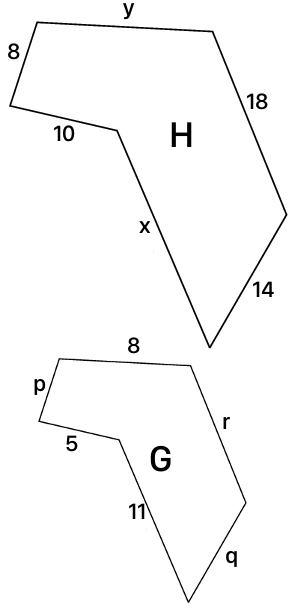1. What is the scale factor ( $k$)?

There is only one pair of matching sides where both measurements are given, namely 5 units and 10 units.

\begin{align} k & = \frac{\text{length of side shape } H} {\text{length of side shape } G} \\ & = \frac{10}{5}\\ & = 2 \end{align}
1. Write down, using the scale factor, the values of $x$, $y$, $p$, $q$ and $r$.

The measurements in polygon $H$ are double the measurements in polygon $G$. The measurements in polygon $G$ are half the measurements in polygon $H$.

$x$ = $2 \times 11$ = 22 units

$y$ = $2 \times 8$ = 16 units

$p$ = $8 \times \frac{1}{2}$ = 4 units

$q$ = $14 \times \frac{1}{2}$ = 7 units

$r$ = 18 $\times \frac{1}{2}$ = 9 units

### Using scale factors to calculate area and volume

You have already seen that you can use a scale factor to find different lengths in two similar shapes.

You will now investigate what will happen if you calculate the areas and volumes of shapes and objects that are enlarged.

### Worked example 9.3: Working with scale factor and area

Two similar right-angled triangles are given. $\triangle DEF$ is an enlargement of $\triangle BAC$.

The scale factor is 2.Calculate the areas of the two triangles and compare the answers.

Use the value of $k$ in your comparison.

1. Step 1: Calculate the area of the given triangle ( $\triangle BAC$).

\begin{align} \text{Area } \triangle BAC & = \frac{1}{2} \times b \times h\\ &= \frac{1}{2} \times 16 \times 12\\ &= \text{96 cm}^{2} \\ \end{align}
2. Step 2: Calculate the area of the new triangle ( $\triangle DEF$).

\begin{align} \text{Area } \triangle DEF& = \frac{1}{2} \times b \times h\\ &= \frac{1}{2} \times 32 \times 24\\ &= \text{384 cm}^{2} \\ \end{align}
3. Step 3: Compare the two answers.

Or: $\text{384 cm}^{2}$= $4 \times \text{96 cm}^{2}$

We can write:

\begin{align} \text{Area of new triangle} & = 4 \times \text{area of given triangle} \\ & = 2^2 \times \text{area of given triangle} \\ \end{align}

This worked example shows an important relationship. If a shape is enlarged by a scale factor $k$, then the area of the new shape will be $k^{2}$ times greater than the area of the given shape.

When we calculate area, we use two dimensions to determine the area of a shape (length $\times$ breadth, or side $\times$ side, for example). If the scale factor is $k$, then each one of these two dimensions must be multiplied by $k$ to find the dimensions for the new shape.

$\therefore$ Area of the new shape = $k^{2} \times$ area of given shape

### Worked example 9.4: Working with scale factor and volume

Two cuboids are given. Cuboid $T$ is an enlargement of cuboid $L$. The scale factor is 2.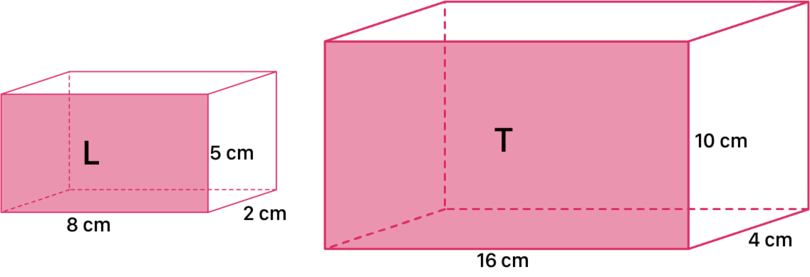Calculate the volumes of the two cuboids and compare the answers.

Use the value of $k$ in your comparison.

1. Step 1: Calculate the volume of the given cuboid ( $L$).

\begin{align} \text{Volume of cuboid } L& = l \times b \times h\\ &= 8 \times 2 \times 5\\ &= \text{80 cm}^{3} \\ \end{align}
2. Step 2: Calculate the volume of the new cuboid ( $T$).

\begin{align} \text{Volume of cuboid } T& = l \times b \times h\\ &= 16 \times 4 \times 10\\ &= \text{640 cm}^{3} \\ \end{align}
3. Step 3: Compare the two answers.

We can write:

\begin{align} \text{Volume of new cuboid} & = 8 \times \text{volume of given cuboid} \\ & = 2^{3} \times \text{volume of given cuboid} \\ \end{align}

This worked example shows another important relationship.

If an object is enlarged by a scale factor $k$, then the volume of the new object will be $k^{3}$ times greater than the volume of the given object.

When we calculate volume, we use three dimensions to determine the volume of an object. Therefore, if the scale factor is $k$, each of these three dimensions must be multiplied by $k$ to find the dimensions for the new object.

$\therefore$ Volume of the new object = $k^{3} \times$ volume of given object

We can summarise the effect of an enlargement using a scale factor of $k$ as follows:

• Length of the new line segment = $k \times$ length of given line segment
• Area of the new shape = $k^{2} \times$ area of given shape
• Volume of the new object = $k^{3} \times$ volume of given object.

We can express each of these relationships as a fraction:

We can also express these relationships as ratios:

\begin{align} \text{length of given line segment } &: \text{length of new line segment}\\ = 1 & : k\\ \end{align} \begin{align} \text{area of given shape } & : \text{area of new shape}\\ = 1 & : k^{2} \\ \end{align} \begin{align} \text{volume of given object }& : \text{volume of new object}\\ = 1 & : k^{3} \\ \end{align}

### Exercise 9.5: Work with scale factor, area, and volume

1. Two similar squares, $E$ and $F$ are given. Consider the diagrams and answer the questions that follow.1. What is the scale factor used to enlarge square $E$?
\begin{align} k & = \frac{\text{side of new square } F} {\text{side of given square } E} \\ & = \frac{20}{4}\\ & =5 \end{align}

The scale factor is 5.

1. Calculate the areas of the two squares and write down a relationship between the two answers.

Area square $E$:

\begin{align} \text{Area}_{E} & = s \times s \\ & = 4 \times 4 \\ & = \text{16 cm}^{2} \\ \end{align}

Area square $F$:

\begin{align} \text{Area}_{F} & = s \times s \\ & = 20 \times 20 \\ & = \text{400 cm}^{2} \\ \end{align}

Relationship between the two areas:

Therefore, we can state that: $\text{400 cm}^{2} = 25 \times \text{16 cm}^{2}$

The relationship is: area square $F$ = $25 \times$ area square $E$

$\therefore$ area square $F$ = $5^{2} \times$ area square $E$

2. Consider the following rectangles. Rectangle $N$ is an enlargement of rectangle $M$.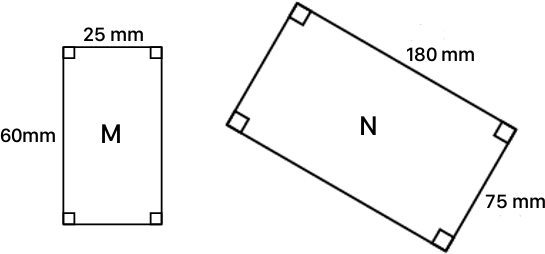What is the relationship between the areas of the two rectangles? (Show all your calculations.)

Find the scale factor first:

\begin{align} k & = \frac{\text{longer side of new shape } N} {\text{longer side of given shape } M} \\ & = \frac{180}{60} \\ & =3 \end{align}

Area of given rectangle:

\begin{align} \text{Area}_{\text{M}} & = l \times b \\ & = 60 \times 25 \\ & = \text{1,500 mm}^{2} \\ \end{align}

Area of new rectangle:

\begin{align} \text{Area}_{\text{N}} & = l \times b \\ & = 180 \times 75 \\ & = \text{13,500 mm}^{2} \\ \end{align}

Relationship between the two areas:

Therefore, we can state that: $\text{13,500 mm} = 9 \times \text{1,500 mm}^{2}$

The relationship is: Area rectangle $N$ = $9 \times$ area rectangle $M$

$\therefore$ area rectangle $N$ = $3^{2} \times$ area rectangle $M$

3. In the diagram below, $\triangle PQR$ is an enlargement of $\triangle DFE$.What is the relationship between the areas of the two triangles? (Show all your calculations.)

Find scale factor first:

\begin{align} k & = \frac{PR}{DE} \\ & = \frac{22}{11} \\ & = 2 \end{align}

Area of given triangle ( $\triangle DFE$):

\begin{align} \text{Area}_{\text{triangle}} & = \frac{1}{2} \times b \times h \\ & = \frac{1}{2} \times 11 \times 5 \\ & = \text{27.5 cm}^{2} \\ \end{align}

Area of new triangle ( $\triangle PQR$):

\begin{align} \text{Area}_{\text{triangle}} & = \frac{1}{2} \times b \times h \\ & = \frac{1}{2} \times 22 \times 10 \\ & = \text{110 cm}^{2} \\ \end{align}

Relationship between the two areas:

\begin{align} \frac{\text{110 cm}^{2}} {\text{27.5 cm}^{2}} & = \frac{110 \times 10}{27.5 \times 10} \\ & = \frac{1,100}{275} \\ & = 4\\ \end{align}

Therefore, we can state that: $\text{110 cm}^{2} = 4 \times \text{27.5 cm}^{2}$

The relationship is: Area $\triangle PQR$ = $4 \times$ area $\triangle DFE$

$\therefore$ Area $\triangle PQR$ = $2^{2} \times$ area $\triangle DFE$

4. Objects $Q$ and $R$ are two cuboids. Cuboid $R$ is an enlargement of cuboid $Q$.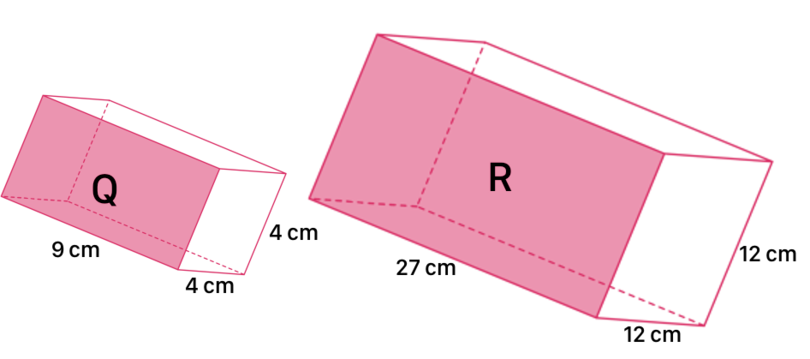What is the relationship between the volumes of the two cuboids? Write the answer as a ratio in the form "Volume cuboid $Q$ : Volume cuboid $R$".

Find the scale factor first:

\begin{align} k & = \frac{\text{longest side cuboid } R}{\text{longest side cuboid } Q} \\ & = \frac{27}{9}\\ & = 3 \end{align}

Volume of cuboid $Q$:

\begin{align} \text{Volume of cuboid } Q& = l \times b \times h\\ &= 4 \times 4 \times 9\\ &= \text{144 cm}^{3} \\ \end{align}

Volume of cuboid $R$:

\begin{align} \text{Volume of cuboid } R& = l \times b \times h\\ &= 12 \times 12 \times 27\\ &= \text{3,888 cm}^{3} \\ \end{align}

Ratio:

\begin{align} \text{Volume cuboid } Q & : \text{Volume cuboid }R\\ = 144& : 3,888\\ = 1& : 27\\ = 1 & : 3^{3}\\ \end{align}
5. Objects $J$ and $K$ shown below are cubes. Cube $K$ is an enlargement of cube $J$, where $k = 4$.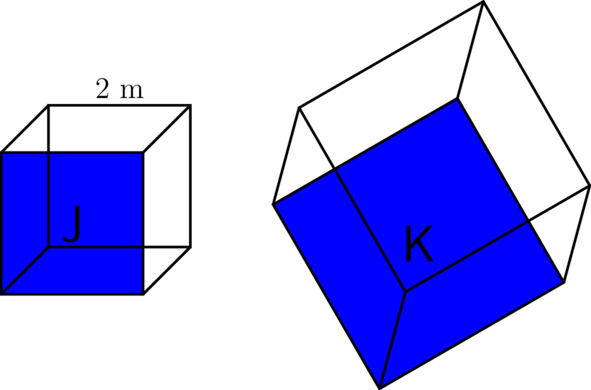1. Calculate the length of one side of cube $K$.
\begin{align} \text{Length of side cube } K & = k \times \text{length of side cube } J\\ &= 4 \times 2\\ &= \text{8 m}\\ \end{align}
1. Calculate the area of one face of cube $K$.

The face of a cube is a square. $\text{Area}_{\text{square}} = s \times s$

\begin{align} \text{Area of face cube } K& = k^{2} \times \text{area of face cube } J\\ &= 4^{2} \times s \times s\\ &= 16 \times 2 \times 2\\ &= \text{64 m}^{2} \\ \end{align}
1. Calculate the volume of cube $K$.
\begin{align} \text{Volume of cube }K & = k^{3} \times \text{volume of cube } J\\ &= 4^{3} \times s \times s \times s\\ &= 64 \times 2 \times 2 \times 2 \\ &= \text{512 m}^{2} \\ \end{align}

## 9.3 Practical applications

Using similar shapes and scale factors can be very helpful in solving problems in our daily lives. In this section you will have the opportunity to apply all the knowledge that you have gained in this chapter.

### Worked example 9.5: Using the scale factor to solve a problem

Oladapo wants to find out the height of a lamp post. Oladapo is 1.5 m tall. The length of Oladapo's shadow changes throughout the day.

Oladapo stands in the shadow of the lamp post, so that his shadow and the lamp post's shadow are in a straight line. The shadows of the lamp post and of Oladapo form triangles, as shown in the diagram below.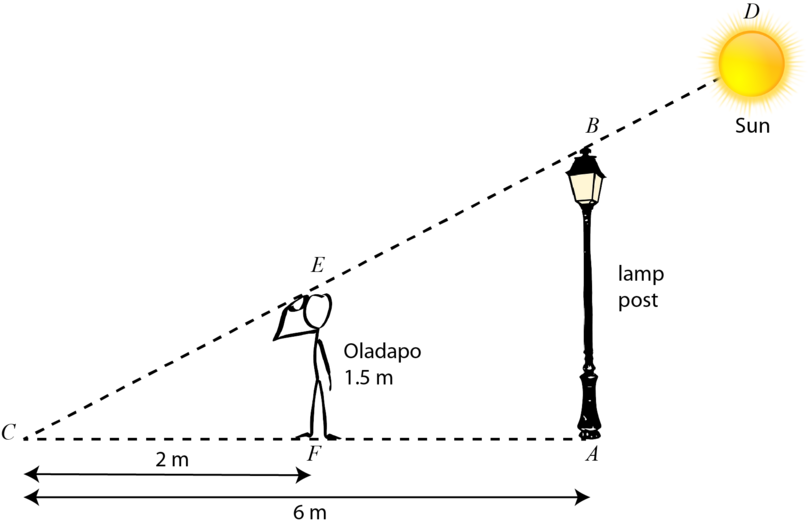Oladapo's shadow is 2 m long and the the shadow of the lamp post is 6 m long.

Draw a diagram showing the triangle formed by Oladapo and his shadow, and a separate diagram showing the lamp post and its shadow. Use your diagrams and find the scale factor of the two trianges to help Oladapo work out the height of the lamp post.

1. Step 1: Draw two separate triangles and fill in all the given information.2. Step 2: Find the scale factor.

$\triangle BAC$ is an enlargement of $\triangle EFC$.

\begin{align} k & = \frac {CA}{CF} \\ & = \frac{6}{2} \\ &= 3\\ \end{align}
3. Step 3: Calculate the height of the lamp post.

The lamp post is represented by $BA$ in the diagram.

We have Oladapo's height, which is EF in the diagram, and we now have the scale factor, so we can calculate the height of the lamp post.

\begin{align} BA& = k \times EF\\ &= 3 \times \text{1.5 m}\\ &= \text{4.5 m}\\ \end{align}
4. Step 4: Give your answer using a full sentence, and include the correct unit of measurement.

The height of the lamp post is 4.5 m.

In real-life questions, as in the example above, we assume that vertical objects like lamp posts and human beings are perpendicular to the ground. We also assume that the ground is perfectly horizontal.

### Exercise 9.6: Use similar shapes and the scale factor to solve problems

1. Ndidi wants to find out the height of a tree. She is 1.6 m tall. The length of Ndidi's shadow changes throughout the day.

At a certain time, Ndidi's shadow is 3 m long and the shadow of the tree is 6 m long.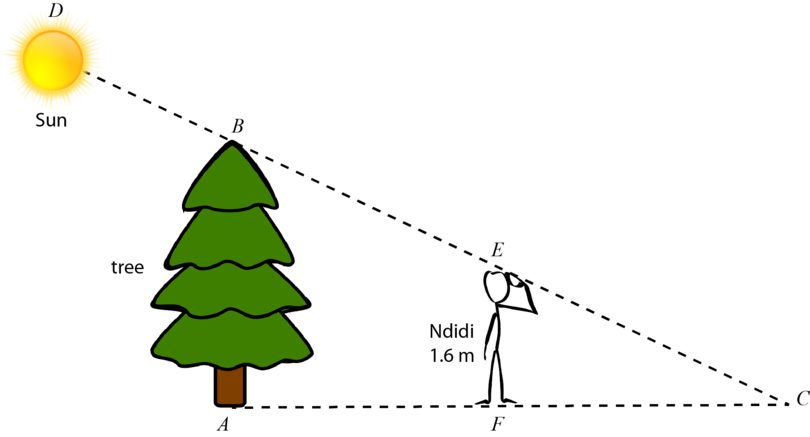Help Ndidi to work out the height of the tree.

Redraw the diagram with the two triangles separate from each other.

The two triangles with all the given information are shown below.$\triangle BAC$ is an enlargement of $\triangle EFC$.

\begin{align} k & = \frac {AC}{FC} \\ & = \frac{6}{3} \\ &= 2\\ \end{align}

The tree is represented by $BA$ on the diagram.

\begin{align} BA& = k \times EF\\ &= 2 \times \text{1.6 m}\\ &= \text{3.2 m}\\ \end{align}

The tree is 3.2 m high.

2. Complete the table below.

$k$ length of given side length of new side
1.5 30 mm
7 371 cm
28.75 m 287.5 m
$k$ length of given side length of new side
1.5 30 mm 1.5 $\times$ 30 = 45 mm
7 53 cm 7 $\times$ 53 = 371 cm
10 28.75 m 10 $\times$ 28.75 = 287.5 m
3. $PQRS$ is a trapezium with measurements (in cm) as shown in the diagram below.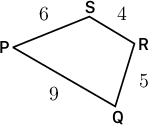Calculate the perimeter of the enlargement of $PQRS$ if a scale factor of 2.25 is used.

\begin{align} \text{Perimeter}_{\text{PQRS}}& = \text{sum of sides}\\ & = 9 + 5 + 4 + 6\\ &= \text{24 cm}\\ \end{align} \begin{align} \text{Perimeter}_{\text{new shape}}& = k \times \text{perimeter of given shape} \\ & = 2.25 \times \text{24 cm}\\ &= \text{54 cm}\\ \end{align}

OR

Calculate the length of each new side first.

If you do not have a diagram of the new shape with new labels for the sides, you can use the prime symbol ( $'$) to show that you are working with the sides of the new shape. So $P'Q'$ is the side of the new shape that corresponds with $PQ$ in the given shape.

\begin{align} \text{Perimeter of new shape} & = 20.25 + 11.25 + 9 + 13.5\\ &= \text{54 cm} \\ \end{align}
4. Complete the table below.

$k$ area of given shape area of new shape
4 $\text{5 mm}^{2}$
6 $\text{1,080 cm}^{2}$
$\text{2 m}^{2}$ $\text{162 m}^{2}$
$k$ area of given shape area of new shape
4 $\text{5 mm}^{2}$ $4^{2} \times 5$ = $\text{80 mm}^{2}$
6 $\text{30 cm}^{2}$ $6^{2} \times 30$ = $\text{1,080 cm}^{2}$
9 $\text{2 m}^{2}$ $9^{2} \times 2$ = $\text{162 m}^{2}$
5. Faruq is designing a pattern to decorate a wall outside a shop. The pattern consists of two equal squares and a rectangle in the middle.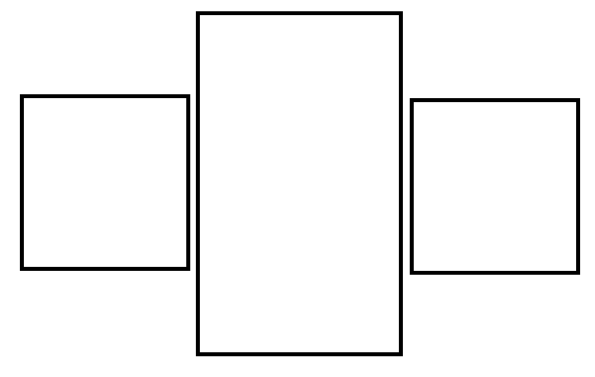The area of one of the squares is $\text{9 cm}^{2}$ and the area of the rectangle is $\text{21 cm}^{2}$.

Calculate the total area of the three shapes if the design is enlarged by a scale factor of 5.

Calculate total area of given shapes:

\begin{align} \text{Total area} & = 9 + 21 + 9 \\ &= \text{39 cm}^{2} \\ \end{align}

Calculate area of new shapes:

\begin{align} \text{Area of new shapes} &= k^{2} \times \text{area of given shapes}\\ &= 5^{2} \times 39 \\ &= 25 \times 39 \\ &= \text{975 cm}^{2} \\ \end{align}
6. Complete the table below.

$k$ volume of given object volume of new object
2 $\text{20 mm}^{3}$
3 $3^{3} \times 4$ = $\text{108 cm}^{3}$
$\text{3 m}^{3}$ $5^{3} \times 3$ = $\text{375 m}^{3}$
$k$ volume of given object volume of new object
2 $\text{20 mm}^{3}$ $2^{3} \times 20$ = $\text{160 mm}^{3}$
3 $\text{4 cm}^{3}$ $3^{3} \times 4$ = $\text{108 cm}^{3}$
5 $\text{3 m}^{3}$ $5^{3} \times 3$ = $\text{375 m}^{3}$
7. Chike is working with the diagram of a cuboid that is given below.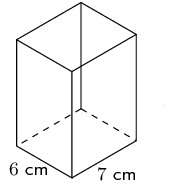Unfortunately the diagram does not show the height of the cuboid. The only information that Chike has is this:

If the cuboid is enlarged by a factor of 2, the volume of the cuboid will be $3,360 \text{ cm}^{3}$.

Help Chike to calculate the height of the cuboid in the diagram.

Chike can use the relationship of the two volumes: Volume of new cuboid = $k^{3} \times$ volume of given cuboid

This is an equation. First, Chike must calculate the volume of the given cuboid, and Chike can solve this equation to do that.

\begin{align} k^{3} \times \text{volume of given cuboid} & = \text{volume of new cuboid}\\ 2^{3} \times \text{volume of given cuboid} & = 3,360 \\ 8 \times \text{volume of given cuboid} &= 3,360\\ \therefore \text{volume of given cuboid} & = 3,360 \div 8 \\ & = \text{420 cm}^{3} \\ \end{align}

Now that Chike has the volume of the given cuboid, $\text{420 cm}^{3}$, he can solve the equation for volume to find the height, which is the unknown.

\begin{align} l \times b \times h &= \text{volume}\\ 7 \times 6 \times h &= 420\\ 42 \times h &= 420\\ h &= 420 \div 42\\ \therefore h &= \text{10 cm} \\ \end{align}

The height of the cuboid in the diagram is 10 cm.

8. The cube shown below has a volume of $\text{27 cm}^{3}$.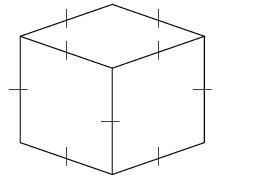Calculate the dimensions of an enlarged cube that has a volume of $1,728\text{ cm}^{3}$.

Find the value of $k$ first:

\begin{align} k^{3} & = \frac{\text{volume of new cube}}{\text{volume of given cube}} \\ &= \frac {1,728}{27} \\ &= 64\\ \end{align}

From $k^{3}$ you need to find the value of $k$. You can find the prime factors of 64:

There are 6 factors here, and you need to have 3 factors:

$4 \times 4 \times 4 = 64$, so $k = 4$

The given cube was enlarged by a scale factor of 4.

It is given that the volume of the given cube = $\text{27 cm}^{3}$.

The formula for the volume of a cube is $V = s \times s \times s = s^3$.

So you also need to have 3 factors of 27. The prime factors of 27 are $3 \times 3 \times 3 = 27$.

Therefore, each side of the given cube = $\text{3 cm}$.

\begin{align} \text{Side of new cube} & = k \times \text{side of given cube} \\ &= 4 \times 3 \\ &= \text{12 cm} \\ \end{align}

The dimensions of the enlarged cube are $\text{12} \times \text{12} \times \text{12 cm}$.

## 9.4 Summary

• In Mathematics, two shapes are similar if:
• their matching sides are in proportion, and
• their matching angles are equal.
• The sides of two shapes are in proportion if all of the sides of the given shape have been multiplied by the same number to get the sides of the new shape. This number is called the scale factor.
• We can use the variable $k$ to represent the scale factor.
• An enlargement of a diagram, shape or object is a copy of the original in which everything is made larger, keeping the same proportions.
• We can summarise the effect of an enlargement using a scale factor of $k$ as follows:
• Length of the new line segment = $k \times$ length of given line segment
• Area of the new shape = $k^{2} \times$ area of given shape
• Volume of the new object = $k^{3} \times$ volume of given object
• We can express each of these relationships as a fraction:

• We can also express these relationships as ratios:
\begin{align} \text{length of given line segment } &: \text{length of new line segment}\\ = 1 &: k\\ \end{align} \begin{align} \text{area of given shape }& : \text{area of new shape}\\ = 1 & : k^{2} \\ \end{align} \begin{align} \text{volume of given object }& : \text{volume of new object}\\ = 1 & : k^{3} \\ \end{align}